# GMAT Math : DSQ: Calculating the perimeter of an equilateral triangle

## Example Questions

2 Next →

### Example Question #101 : Triangles

Which, if either, of equilateral triangles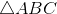and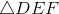, has the greater perimeter?

Statement 1: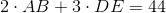Statement 2: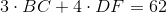Statement 2 ALONE is sufficient to answer the question, but Statement 1 ALONE is NOT sufficient to answer the question.

EITHER statement ALONE is sufficient to answer the question.

Statement 1 ALONE is sufficient to answer the question, but Statement 2 ALONE is NOT sufficient to answer the question.

BOTH statements TOGETHER are sufficient to answer the question, but NEITHER statement ALONE is sufficient to answer the question.

BOTH statements TOGETHER are insufficient to answer the question.

BOTH statements TOGETHER are sufficient to answer the question, but NEITHER statement ALONE is sufficient to answer the question.

Explanation:

If we letandbe the sidelengths ofand, respectively; the statements can be rewritten as:

Statement 1: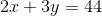Statement 2: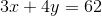Since the perimeter of an equilateral triangle is three times its common sidelength, comparison of the lengths of the sides is all that is necessary to determine which triangle, has the greater perimeter. The question can therefore be reduced to asking which ofand, if either, is greater.

Statement 1 alone is not sufficient to yield an answer:

Case 1: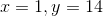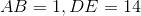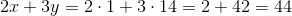Case 2: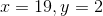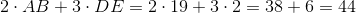Both cases satisfy Statement 1, but in the first case,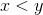, meaning thathas greater sidelength and perimeter than, and in the second case,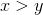, meaning the reverse. By a similar argument, Statement 2 is insufficient.

Now assume both statements to be true. The two equations together comprise a system of equations:Multiply the first equation by 3 and the second by, then add: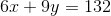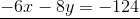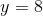Now substitute back: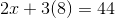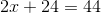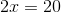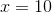has the greater sidelength and, consequently, the greater perimeter.

### Example Question #102 : Triangles

Given an equilateral triangleand a right trianglewith right angle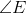, which has the greater perimeter?

Statement 1: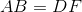Statement 2: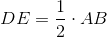Statement 1 ALONE is sufficient to answer the question, but Statement 2 ALONE is NOT sufficient to answer the question.

EITHER statement ALONE is sufficient to answer the question.

BOTH statements TOGETHER are insufficient to answer the question.

Statement 2 ALONE is sufficient to answer the question, but Statement 1 ALONE is NOT sufficient to answer the question.

BOTH statements TOGETHER are sufficient to answer the question, but NEITHER statement ALONE is sufficient to answer the question.

Statement 1 ALONE is sufficient to answer the question, but Statement 2 ALONE is NOT sufficient to answer the question.

Explanation:

Assume Statement 1 alone. Since inis the right angle,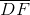is the hypotenuse, which is longer than either of the two legs -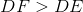and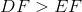. Since, in equilateral triangle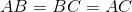, it follows that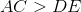and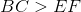. Consequently,

andhas the greater perimeter.

Statement 2 alone gives insufficient information, since we know nothing about the second leg or the hypotenuse of. We see this by examining these two cases.

Case 1: Letbe an equilateral triangle of sidelength 6, and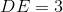in. If second leg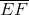ofhas length 4, then the triangleis a 3-4-5 triangle, with hypotenusehaving length 5. The perimeter ofis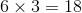, and the perimeter ofis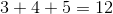has the greater perimeter.

Case 2: Letbe an equilateral triangle of sidelength 6, andin. If second legofhas length 20,has the greater perimeter on the basis of one side alone.

### Example Question #103 : Triangles

Find the perimeter of the equilateral triangle.

1. A side measures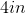.
2. The area is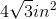.

Both statements taken together are sufficient to answer the question, but neither statement alone is sufficient.

Each statement alone is sufficient to answer the question.

Statement 2 alone is sufficient, but statement 1 alone is not sufficient to answer the question.

Statements 1 and 2 are not sufficient, and additional data is needed to answer the question.

Statement 1 alone is sufficient, but statement 2 alone is not sufficient to answer the question.

Each statement alone is sufficient to answer the question.

Explanation:

Recall the formula for perimeter of a triangle.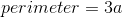whererepresents the side length of the triangle

Statement 1: We're given the length of the side so all we need to do is plug this value into the equation.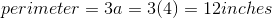Statement 2: We're given the area so we first need to solve for the side length.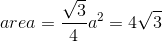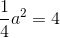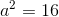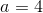Now we can plug the value into the equation, like we did in Statement 1.2 Next →

Tired of practice problems?

Try live online GMAT prep today.